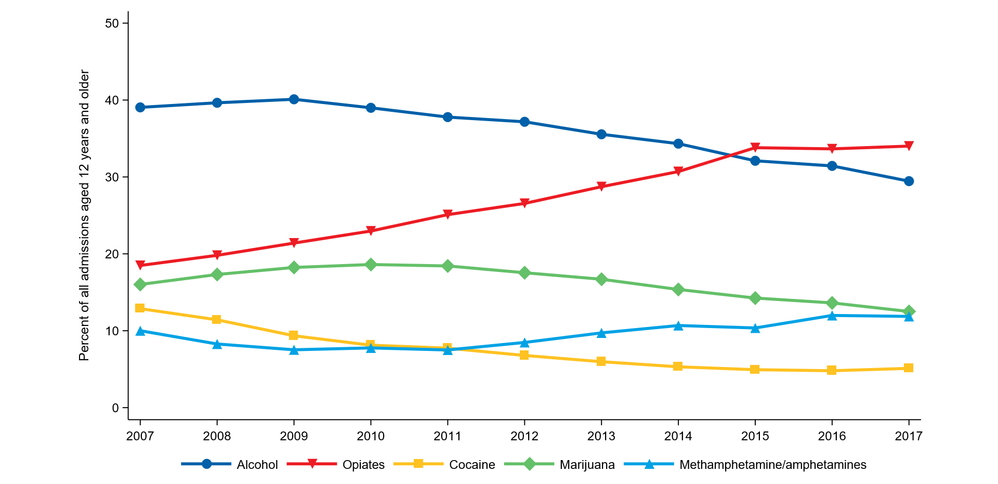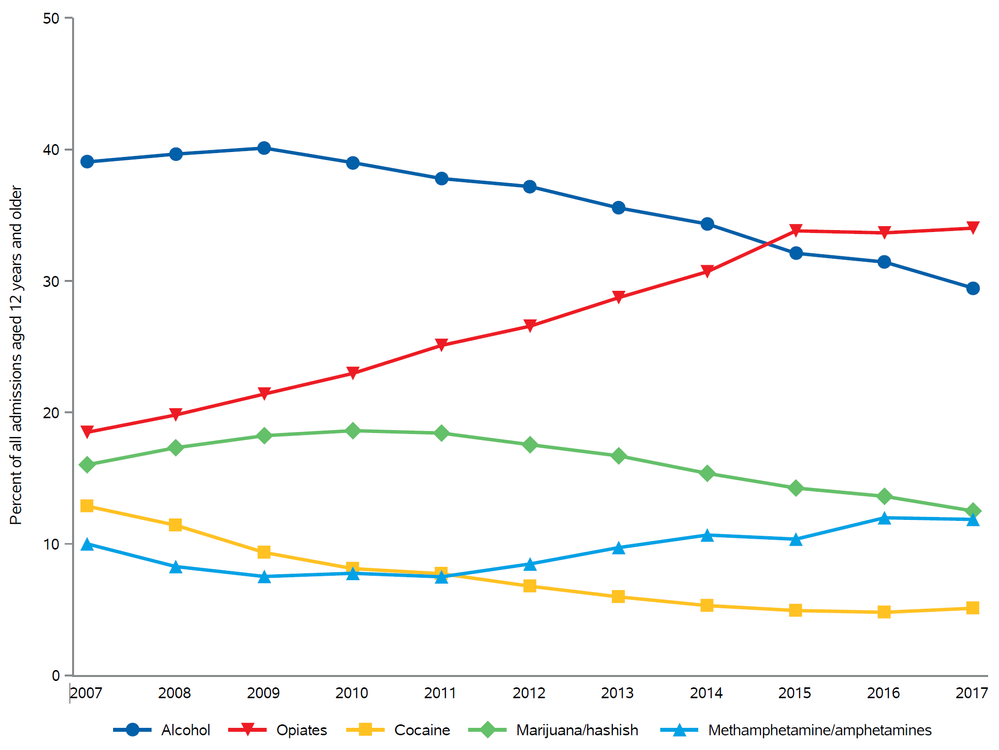## Increase spacing in SGPLOT legend

I have created the graph below with SGPLOT and need to increase the spacing between the legend markers and the value labels. I can't find an argument for the keylegend statement that will allow me to do this. Any suggestions?1 ACCEPTED SOLUTION

Accepted Solutions

## Re: Increase spacing in SGPLOT legend

I assigned labels however, I left your code 99% intact but wrapped inside an absolute layout (could use gridded also) and specified the dimension that you can change.  You did not specify the output format so I picked pdf``````data prim_sub_use; set xl.sheet1;
label
Alcohol	='^_^_Alcohol^_'
Opiates	='^_^_Opiates^_'
Cocaine	='^_^_Cocaine^_'
Marijuana='^_^_Marijuana/hashish^_'
Methamphetamine_amphetamines='^_Methamphetamine/amphetamines^_'
;
run;
ods escapechar='^';
ods _all_ close;
options nonumber nodate orientation=landscape papersize=letter nocenter
leftmargin=.5in rightmargin=.5in topmargin=.5in bottommargin=.5in;
ods pdf file="~/img/abuse.pdf" dpi=300 notoc;
ods layout absolute;
ods region x=0in y=0in width=10in;
ods graphics on /noborder height=7.5in width=10in scale=on;
proc sgplot data=prim_sub_use noborder nowall
description='Primary substance use at admission, 2007-2017';
series x=Year y=Alcohol/markers markerattrs=(symbol=CircleFilled
color='#0060A9' size=12) lineattrs=(color='#0060A9' thickness=3);
series x=Year y=Opiates/markers markerattrs=(symbol=TriangleDownFilled size=12
color='#ed1c24') lineattrs=(color='#ed1c24' thickness=3 pattern=solid);
series x=Year y=Cocaine/markers markerattrs=(symbol=SquareFilled
color='#ffc222' size=12) lineattrs=(color='#ffc222' thickness=3
pattern=solid);
series x=Year y=Marijuana/markers markerattrs=(symbol=DiamondFilled
color='#64c069' size=12) lineattrs=(color='#64c069' thickness=3
pattern=solid) ; * legendlabel="Marijuana/hashish";
series x=Year y=Methamphetamine_amphetamines/markers
markerattrs=(symbol=TriangleFilled size=12 color='#00a2e5')
lineattrs=(color='#00a2e5' thickness=3 pattern=solid);
yaxis label='Percent of all admissions aged 12 years and older' VALUES=(0 TO 50 BY 10) offsetmax=0 offsetmin=0;
xaxis label='Admission Year' VALUES=(2007 TO 2017 BY 1) display=(nolabel 		noticks);
keylegend/noborder linelength=30;
run;
ods graphics off;
ods layout end;
ods pdf close;
ods _ALL_ CLOSE;``````

19 REPLIES 19

## Re: Increase spacing in SGPLOT legend

I don't know. I don't remember anything like that. The first thing I would try is adding one or more nonbreaking spaces to the end of the drug name in a DATA step before you run sgplot.

drug = trim(drug) || 'A0'x;

## Re: Increase spacing in SGPLOT legend

That syntax deleted the numeric values of my variables, strange.

## Re: Increase spacing in SGPLOT legend

If you are using a formatted numeric variable to get your drug names, then you will need to modify the approach accordingly.

## Re: Increase spacing in SGPLOT legend

Yes I am using a separate numeric variable for each series on the graph.

## Re: Increase spacing in SGPLOT legend

If your variables are numeric, then you can do something like the following in a data step:

drug2='A0A0'x||strip(vvalue(drug))||'A0A0'x;

Where the number of A0 that you repeat will be the number of non-breaking spaces you add to the value. You would then group by DRUG2 instead of drug.

## Re: Increase spacing in SGPLOT legend

I might be tempted to try something like

Keylegend / across=3 down=2;

and maybe play around with the LINELENGTH option to see about making the length of the lines shorter.

## Re: Increase spacing in SGPLOT legend

That may be an option, but I'd really prefer the legend to all be on 1 line if possible.

## Re: Increase spacing in SGPLOT legend

@martyvd wrote:
That may be an option, but I'd really prefer the legend to all be on 1 line if possible.

There is only so much space available. If the values are long enough there just is not much space left between the lines.

Did you try anything with the LINELENGTH to shorten the displayed line segments in the legend?

## Re: Increase spacing in SGPLOT legend

Yes, decreasing the LINELENGTH value only shortens the line segments, it does not affect the spacing between the line segments and the labels.Jay54
Meteorite | Level 14

## Re: Increase spacing in SGPLOT legend

You may want to consider why you want to increase the space between the
chicklet and the label. As Rick suggested, you would have to add a NBSP
space before each value of group values to add some white space.

Or, if you want to make the legend more bolder, you can use KEYLEGEND
options to adjust the size of the chicklets - FILLASPECT, FILLHEIGHT, SCALE.

## Re: Increase spacing in SGPLOT legend

I tried adding a space to the variables using Jeff's code, but the graph was blank when I used the new variables.Jay54
Meteorite | Level 14

## Re: Increase spacing in SGPLOT legend

The NBSP suggestion originally came from Warren.  Sorry about the incorrect attribution.

Also, my suggested usage of KEYLEGEND options may be more applicable for filled chicklets.

## Re: Increase spacing in SGPLOT legend

I am sure it can be done.  Can you share your code and data (dummied up if it's confidential)

## Re: Increase spacing in SGPLOT legend

``````proc sgplot data=prim_sub_use noborder nowall description='Primary substance use at admission, 2007-2017';
series x=Year y=Alcohol/markers markerattrs=(symbol=CircleFilled color='#0060A9' size=12) lineattrs=(color='#0060A9' thickness=3);
series x=Year y=Opiates/markers markerattrs=(symbol=TriangleDownFilled size=12 color='#ed1c24') lineattrs=(color='#ed1c24' thickness=3 pattern=solid);
series x=Year y=Cocaine/markers markerattrs=(symbol=SquareFilled color='#ffc222' size=12) lineattrs=(color='#ffc222' thickness=3 pattern=solid);
series x=Year y=Marijuana/markers markerattrs=(symbol=DiamondFilled color='#64c069' size=12) lineattrs=(color='#64c069' thickness=3 pattern=solid) legendlabel="Marijuana/hashish";
series x=Year y=Methamphetamine_amphetamines/markers markerattrs=(symbol=TriangleFilled size=12 color='#00a2e5') lineattrs=(color='#00a2e5' thickness=3 pattern=solid);
yaxis label='Percent of all admissions aged 12 years and older' VALUES = (0 TO 50 BY 10) offsetmax=0 offsetmin=0;
xaxis label='Admission Year' VALUES = (2007 TO 2017 BY 1) display=(nolabel noticks);
keylegend/noborder linelength=30;
run;``````

Discussion stats
• 19 replies
• 2477 views
• 1 like
• 6 in conversation